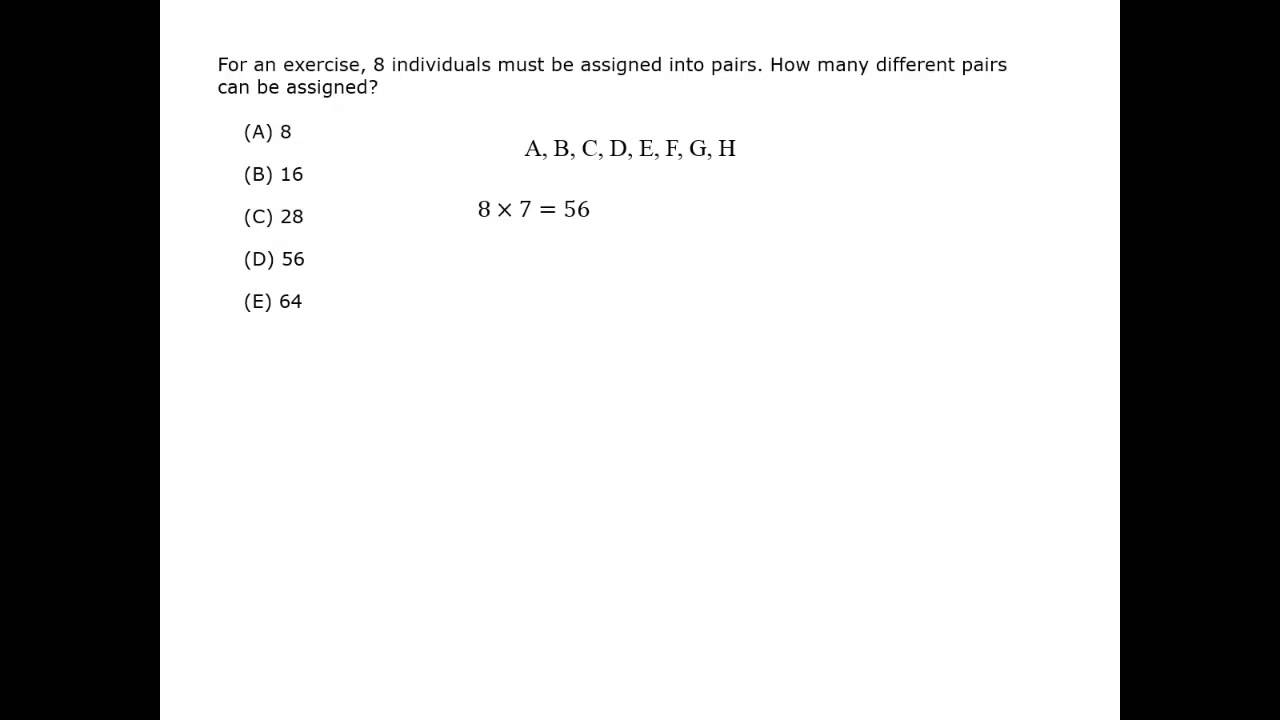Free GMAT Course > GMAT Math Basics > 4. Combinatronics > Introduction to Combinations

Combinations and Permutations

Video Courtesy of (site-affiliate) Kaplan GMAT prep.

## Order is Not Important

Combinations problems are very similar to permutations problems. The key distinction is that, unlike permutations, arrangement or order doesn’t matter for combinations.

A combination is a selection of objects or elements from a group where order is not relevant. Combination questions ask how many selections or subsets there are. For example, if a committee is being selected and there will be a president, vice president, and treasurer, then order matters so it is a permutation problem. But if a committee of three people is being formed without specific roles, then order does not matter and it is a combination problem.

Video Courtesy of (site-affiliate) Kaplan GMAT prep.

## Permutation vs. Combination

The two examples below use very similar dice games, but a slight difference means one uses permutations, and the other uses combinations. The comparison illustrates the difference between permutations and combinations.

permutation
In a game of dice, you roll one red die and one blue die and record the results. If you roll “doubles” (both dice land on the same face), the results aren’t counted and you roll again. How many permutations are possible for one roll?

### Solution

Method 1

1. Figure out how many places there are to fill.
Because there are two dice, there are two places to fill:  ___  ___

2. Figure out how many objects can potentially go into each place.
Since you cannot get the same result (“doubles”) on the second die, there are only 5 possibilities for the second place:    6   5

3. Multiply.
6 × 5 = 30

Method 2

Use the formula.

6 P 2 = \dfrac{6!}{(6 \,-\, 2)!} = \dfrac{6!}{4!} = \dfrac{6 × 5 × 4!}{4!}

= 6 × 5 = 30
Notice that 6! has 4! as a factor.

Method 3

This table shows all of the 36 possibilities for rolling two dice. The doubles on the diagonal are ignored, so for this game there are 30 different permutations.

 (Red, Blue) (Red, Blue) (Red, Blue) (Red, Blue) (Red, Blue) (Red, Blue) (1, 1) (2, 1) (3, 1) (4, 1) (5, 1) (6, 1) (1, 2) (2, 2) (3, 2) (4, 2) (5, 2) (6, 2) (1, 3) (2, 3) (3, 3) (4, 3) (5, 3) (6, 3) (1, 4) (2, 4) (3, 4) (4, 4) (5, 4) (6, 4) (1, 5) (2, 5) (3, 5) (4, 5) (5, 5) (6, 5) (1, 6) (2, 6) (3, 6) (4, 6) (5, 6) (6, 6)
combination
In a game of dice, you roll 2 red dice and record the results. If you roll “doubles” (both dice land on the same face), the results aren’t counted and you roll again. How many combinations are possible for one roll?

### Solution

Since the dice are the same color, order is not important. This is a combinations question.

In the first game, the outcome (5, 2) is different than the outcome (2, 5). In this game, the dice are the same color, so there is no difference between (2, 5) and (5, 2). These two outcomes are counted as only one combination.

In combinations questions, you must eliminate the duplicate results.

This table shows all the 36 possibilities for rolling two dice. The doubles on the diagonal are ignored. Of the remaining possibilities, half are duplicates. There are the 15 different, distinct combinations.

 (Red, Red) (Red, Red) (Red, Red) (Red, Red) (Red, Red) (Red, Red) (1, 1) (2, 1) (3, 1) (4, 1) (5, 1) (6, 1) (1, 2) (2, 2) (3, 2) (4, 2) (5, 2) (6, 2) (1, 3) (2, 3) (3, 3) (4, 3) (5, 3) (6, 3) (1, 4) (2, 4) (3, 4) (4, 4) (5, 4) (6, 4) (1, 5) (2, 5) (3, 5) (4, 5) (5, 5) (6, 5) (1, 6) (2, 6) (3, 6) (4, 6) (5, 6) (6, 6)

## Combinations Formula

The tables above illustrate the logic behind the difference between combinations and permutations.

The formula for combinations follows from this logic.

n C r = \dfrac{\textit{n}!}{\textit{r}! (\textit{n} \,-\, \textit{r})!}

In this formula:

• n stands for the number of distinct objects that you are choosing from.
• r stands for the number of objects being chosen, or the places to fill.
• C stands for combination, and is not an arithmetic part of the equation.
• The exclamation point (!) denotes the factorial of that number.

This formula is very similar to the permutations formula. The only difference is the addition of r! on the bottom. So the steps to find combinations are:

1. Start the problem as if it was a permutations problem.

1. Figure out how many places there are to fill.
2. Figure out how many objects can potentially go into each place.
3. Multiply.

2. Divide the answer by the factorial of the number of places.

#### 800score Tip:

There are always fewer Combinations than Permutations.

Look at the formulas: Using the same values for n and r, the formula for combinations is the formula for permutations divided by r!

Use logic: When results with different orders are counted only once (combinations) then there must be fewer possibilities than when those same results are counted multiple times (permutations).

combination

In a game of dice, you roll 2 dice and record the results. If you roll “doubles,” the results aren’t counted and you roll again. How many combinations are possible for one roll?

### Solution

Solve this again, this time by using the formula.

6 C 2 = \dfrac{6!}{2! (6 \,-\, 2)!} = \dfrac{6!}{2! × 4!}

= \dfrac{6 × 5 × 4!}{2 × 4!} = 3 × 5 = 15

There are 15 combinations.

Notice that there are only 15 combinations while there are 30 permutations. Having order (red and blue dice) increased the number of possibilities.

#### Introduction to Combinations

Best viewed in landscape mode

1 question with a video explanation

100 seconds per question